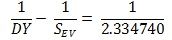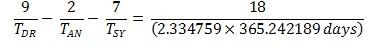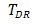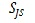## Thursday, November 12, 2015

### A link between the Lunar tidal cycles and the planetary orbital periods of Venus, Earth, Jupiter and Saturn

SUMMARY OF RESULTS:The 2.334740 tropical year lunar tidal period is effectively just the synodic product of the lunar Draconic Year (DY) with the Synodic period of Venus and the Earth.There is a link between the synodic orbital periods of Venus/Earth and Jupiter/Saturn with the Lunar Nodal Cycle (LNC), Lunar Anomalistic Cycle (LAC), and the lunar Draconic Year (DY).

[N.B. all values above are in tropical years]

N.B. In addition, a connection was found between the synodic orbital periods of Venus/Earth and Jupiter/Saturn and the LAC when the time variables were expressed in sidereal years.

http://astroclimateconnection.blogspot.com.au/2015/05/the-six-year-re-alignment-period.html

START OF MAIN POST:

In an earlier post located at:

http://astroclimateconnection.blogspot.com.au/2015/11/two-new-connections-between-planetary.html

A. It was established that if you took the minimum period between the times of maximum change in the tidal stresses acting upon the Earth that are caused by changes in the direction of the lunar tides (i.e. 1.89803 tropical years = 2.0 Draconic years), and amplitude modulate this period by the minimum period between the times of maximum change in tidal stresses acting upon the Earth that are caused by changes in the strength of the lunar tides (i.e. 10.14686 tropical years = 9.0 Full Moon Cycles), you found that the 1.89803 year tidal forcing term is split into a positive and a negative side-lobe, such that:

Positive side-lobe
[10.14686 x 1.89803] / [10.14686 – 1.89803] = 2.3348 tropical yrs = 28.02 months    (1)

Negative side-lobe
[10.14686 x 1.89803] / [10.14686 + 1.89803] = 1.5989 tropical yrs                               (2)

where

10.146856 tropical years = 3706.059873 days = 9.0 Full Moon Cycles (FMC) and 1.0 FMC = 411.78443029 days = 1.127428 tropical years is the synodic beat period between the mean anomalistic month and the mean Synodic month.
1.89803 tropical years = 693.2401518 days = 2.0 Draconic Years and
1.0 Draconic Year = 346.62007588 days = 0.949014 tropical years is the synodic beat period between the between the mean Draconic month and the mean Synodic month.
Equation (1) can be rewritten as:(3)
and equation (2) can be rewritten as:(4)
where= the Draconic month = 27.212221 days= the anomalistic month = 27.554550 days= the Synodic month = 29.5305889 days

and 1.598939 tropical years = 583.999980 days  which differs from the Synodic period of Venus and the Earth by only 1.90 hours.

Adding equations (3) and (4) gives you:(5)

where= 1.598939 tropical years, which differs from the synodic period of Venus and the Earth by only 1.90 hours.

DY = 346.6190478 days = 0.9490115 tropical years,  which differs from the Draconic year by only 1.48 minutes.

Hence, the 2.334740 tropical year lunar tidal period is effectively just the synodic product of the lunar Draconic Year (DY) with the Synodic period of Venus and the Earth.

B.  It was established that:(6)

where= the Synodic period of Jupiter and Saturn = 19.8596 tropical years
LNC = Lunar Nodal Cycle = 18.6000 tropical years
LAC = Lunar anomalistic Cycle = 8.8505 tropical years

which, with the substitution of equation (5), can be rewritten as:(7)

Hence, equation (7) shows that there is a link between the synodic orbital periods of Venus/Earth and Jupiter/Saturn with the Lunar Nodal Cycle (LNC), Lunar Anomalistic Cycle (LAC), and the lunar Draconic Year (DY).